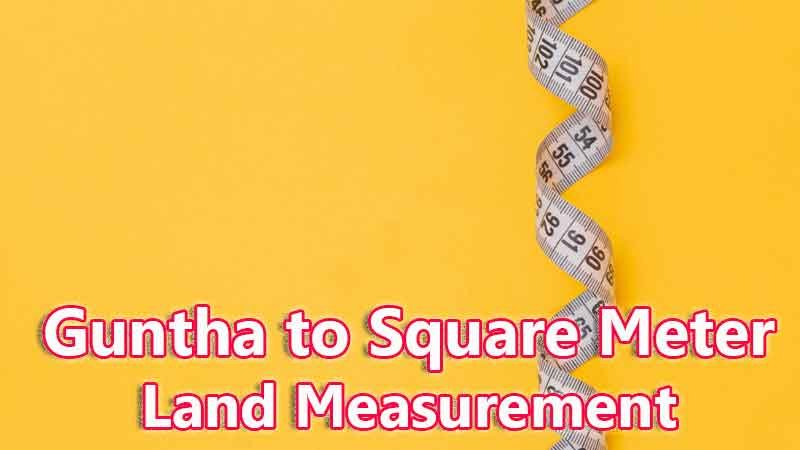# Guntha To Square Meter Converter

Guntha is a traditional land unit used to measure the land Area standardly. Here we are using the guntha to square meter unit value conversion. And guntha also comes under these land area units like Gonda, Kani, and square meter. In West Bengal, One guntha is directly equivalent to 101.1715084681203 square meter.

Input Here

guntha

Output

square meter

1 guntha = 101.1715084681203 square meter
Rounded Value Is (1 guntha = 101 square meter)

## Measurement

Guntha means - a unit of land measurement. This tool provides great calculation over guntha to square meter, 1 guntha to square meter, 1 guntha in square meter, 1 guntha equal to and etc. To convert guntha to square meter, then multiply the unit value by 101.1715084681203. Then, 1 guntha * 101.1715084681203 = 101.1715084681203 square meter. Hence, 1 guntha is equal to 101.1715084681203 square meter.

Square Meter also comes under this land measurement unit and we are comparing values between guntha traditional unit with other side land measurement unit.

## Formula For guntha to square meter Conversion(guntha to square meter formula)

The base formula for this guntha to square meter converter is

square meter = guntha * 101.1715084681203

## How many square meter in a guntha?

Step 1: To Convert 1 guntha to square meter

Step 2: Applying formula square meter = guntha * 101.1715084681203, (i.e) multiply the unit value by 101.1715084681203.

Step 3: Then, square meter = 1 * 101.1715084681203 = 101.1715084681203.

Step 4: Hence, 1 guntha is equal to 101.1715084681203 square meter.Some guntha to square meter Conversion Chart for your reference:

 1 guntha = 101.1715084681203 square meter 2 guntha = 202.3430169362406 square meter 3 guntha = 303.5145254043609 square meter 4 guntha = 404.6860338724812 square meter 5 guntha = 505.85754234060147 square meter 6 guntha = 607.0290508087218 square meter 7 guntha = 708.2005592768421 square meter 8 guntha = 809.3720677449624 square meter 9 guntha = 910.5435762130827 square meter 10 guntha = 1011.7150846812029 square meter

The guntha to square meter conversion chart is above listed for your reference. This chart, however, represents the simple math calculation involved in the guntha to square meter convertion online.

For Example: How much is 1 guntha to square meter

Solution:

= (guntha * 101.1715084681203)

= (1 x 101.1715084681203)

= 101.1715084681203 square meter

For Example: How much is 120 guntha in square meter

Solution:

= (guntha * 101.1715084681203)

= (120 x 101.1715084681203)

= 12140.581016174436 square meter

This formula provides an instant answer for all your questions / People also search:

• 1 guntha to square meter
• 1 guntha is equal to how many square meter
• Formula to convert guntha to square meter online
• How to convert guntha to square meter?
• How much square meter is equal to a one guntha?
• How many square meter in guntha?
• 1 guntha equal to square meter